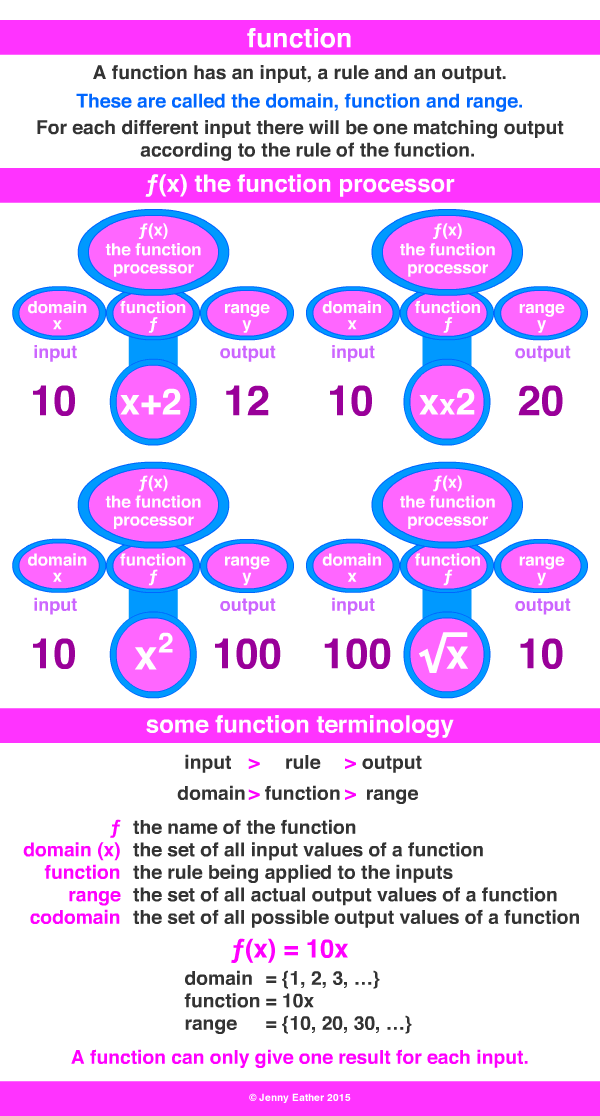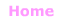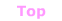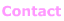Aa Bb Cc Dd Ee Ff Gg Hh Ii Jj Kk Ll Mm Nn Oo Pp Qq Rr Ss Tt Uu Vv Ww Xx Yy Zz

Ff

function

• a mathematical relationship from a set of inputs to a set of outputs.
•  the output value depends on (is a function of) the input value
and each input produces exactly one output.
• the set of all inputs is called the domain,
the set of all possible outputs is the codomain
and the set of actual outputs is the range.

EXAMPLES:© Jenny Eather 2014. All rights reserved.